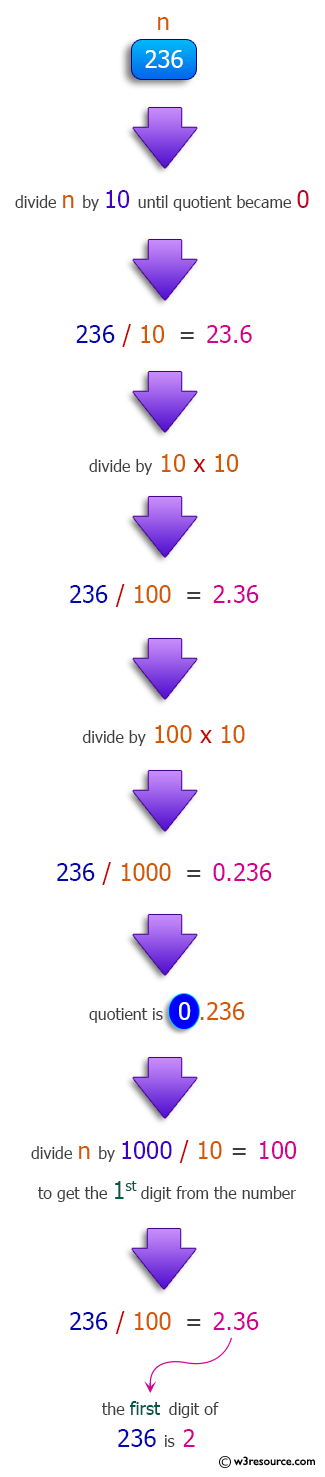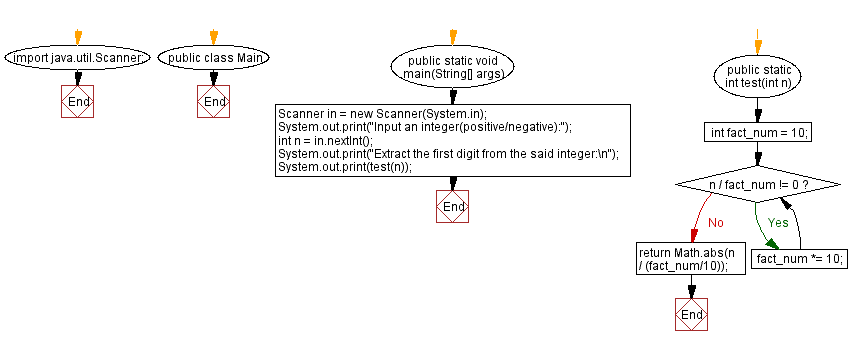﻿ Java: Extract the first digit from an integer# Java Method Exercises: Extract the first digit from an integer

## Java Method: Exercise-20 with Solution

Write a Java method to develop a Java method for extracting the first digit from a positive or negative integer

Pictorial Presentation:Sample data:
(1234) ->1
(-2345) ->2

Sample Solution:

Java Code:

``````import java.util.Scanner;
public class Main {
public static void main(String[] args)
{
Scanner in = new Scanner(System.in);
System.out.print("Input an integer(positive/negative):");
int n = in.nextInt();
System.out.print("Extract the first digit from the said integer:\n");
System.out.print(test(n));
}

public static int test(int n){
int fact_num = 10;
while(n / fact_num != 0){
fact_num *= 10;
}
return Math.abs(n / (fact_num/10));
}
}
```
```

Sample Output:

```Input an integer(positive/negative): 1234
Extract the first digit from the said integer:
1
```

Flowchart :Java Code Editor:

Previous Java Exercise: Accept three integers and return the middle one.
Next Java Exercise: Display the factors of 3 in a given integer

What is the difficulty level of this exercise?

Test your Programming skills with w3resource's quiz.

﻿